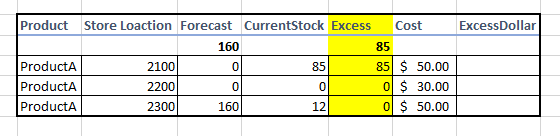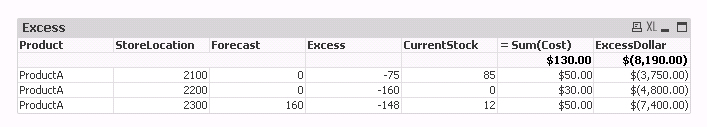# New to QlikView

Discussion board where members can get started with QlikView.

Announcements
Talk to Experts Tuesday, Live Q&A, September 22: Moving from QlikView to Qlik Sense. REGISTER
cancel
Showing results for
Did you mean:
HighlightedContributor II

## Help with IF condition for field totalI will like to calculate the total excess column using the following condition

if sum of currentstock - forecast > 0, excess is currentstock - forecast, else its zero.

As u can see the sum of excess is 85 which is less than the forecast, i want to compare their totals and if excess is less than forecast, i should get a zero as the forecast will eat up the excess.

if the total excess is bigger than the forecast, then the excess with multiply the corresponding cost to give excess dollar. I know its a lot but I will really appreciate your input.

9 Replies
HighlightedChampion III

Can you share some sample data as text rather than image. And your expected output you would want to see?

HighlightedContributor II

I have attached a simple excel that explains what i want to do. Let me know if that clears it up

Thanks

HighlightedSpecialist II

Is this what you are looking for -

HighlightedChampion III

is this what you are looking for? If yes, then add straight table with following dimensions and expression accordingly.Dim:

Product

StoreLocation

Forecast

Excess

expr:

= IF(Sum(TOTAL <Product> CurrentStock) > Sum(TOTAL <Product> Forecast), 0, Sum(TOTAL <StoreLocation> CurrentStock) - Sum(TOTAL <Product> Forecast))

= Sum(Cost)

= Num(fabs(Column(1) * Sum(Cost)), '\$'&'(#,##0.00)')

Change the labels for expressions accordingly.

HighlightedContributor II

thanks for the response, the excess column is returning 0 for everything, i tried it on my dataset and it returns zero all through

HighlightedContributor II

thanks a lot for your response, i tried your solution on my dataset and the excess column returned same value as the currentstock

HighlightedChampion III

Can you try this for excess? If not can you send us the screen shot where you can see the 0's coming

= IF(Sum(TOTAL <Product> CurrentStock) > Sum(TOTAL <Product> Forecast), 0, Sum(TOTAL <StoreLocation, Product> CurrentStock) - Sum(TOTAL <Product> Forecast))

HighlightedContributor IIso i had to blur off some parts but its the same as i showed earlier, the material is the product.

HighlightedChampion III

‌you have lot of other dimensions here we have to look into your actual data set to come up with correct expression. Based on what you sent in your excel it works but if your dataset is different we might need to tweak around. Can you share your qvw file masking data. You can scramble your data using scramble technique. Check here

Preparing examples for Upload - Reduction and Data Scrambling